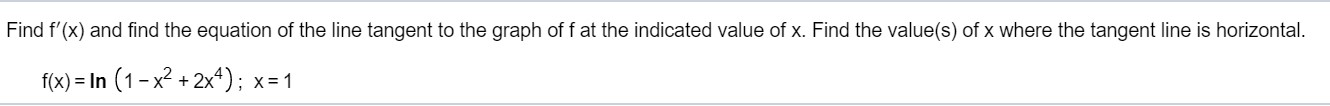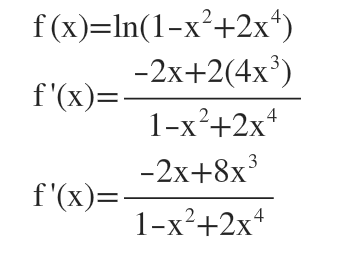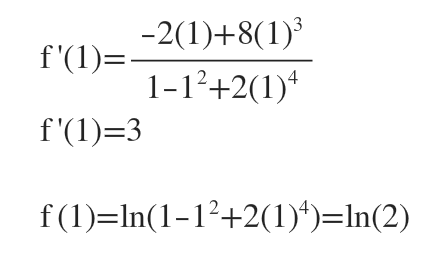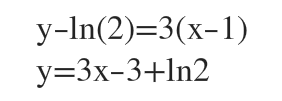# Find f'(x) and find the equation of the line tangent to the graph of f at the indicated value of x. Find the value(s) of x where the tangent line is horizontal.f(x) = In (1-x² + 2x*); x=1

Question
7 viewshelp_outlineImage TranscriptioncloseFind f'(x) and find the equation of the line tangent to the graph of f at the indicated value of x. Find the value(s) of x where the tangent line is horizontal. f(x) = In (1-x² + 2x*); x=1 fullscreen
check_circle

Step 1

We find f'(x) using the chain ruleStep 2

At x=1,We use the point slope formula to find the equation of the tangent...

### Want to see the full answer?

See Solution

#### Want to see this answer and more?

Solutions are written by subject experts who are available 24/7. Questions are typically answered within 1 hour.*

See Solution
*Response times may vary by subject and question.
Tagged in